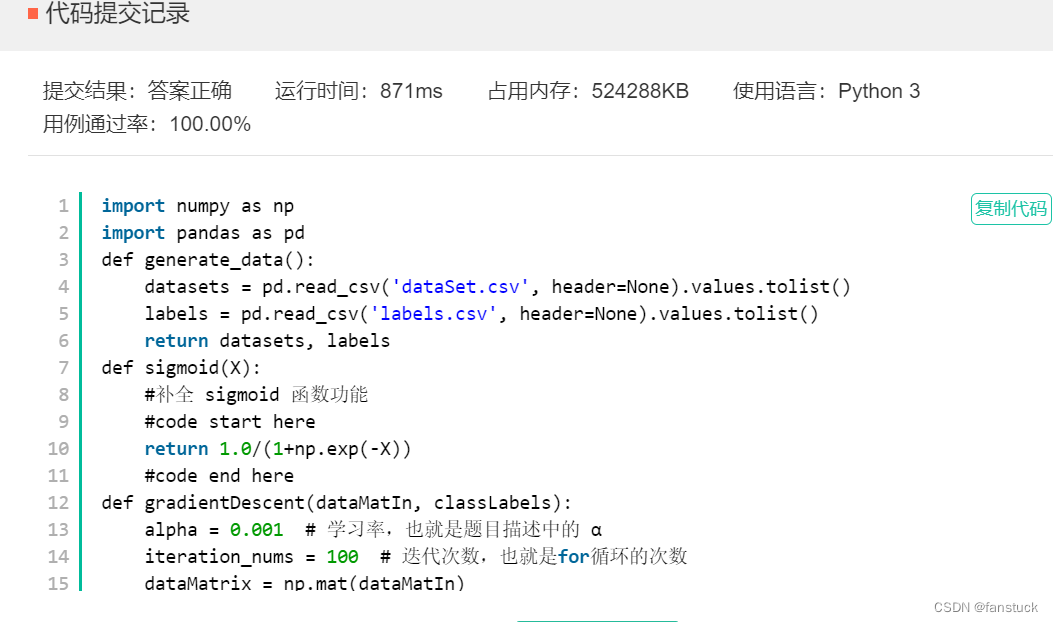# 机器学习实战运用：速刷牛客5道机器学习题目

解答

四、AI4 决策树的生成与训练-信息增益

解答：

五、AI5 使用梯度下降对逻辑回归进行训练

Logistic模型原理详解以及Python项目实现

# 前言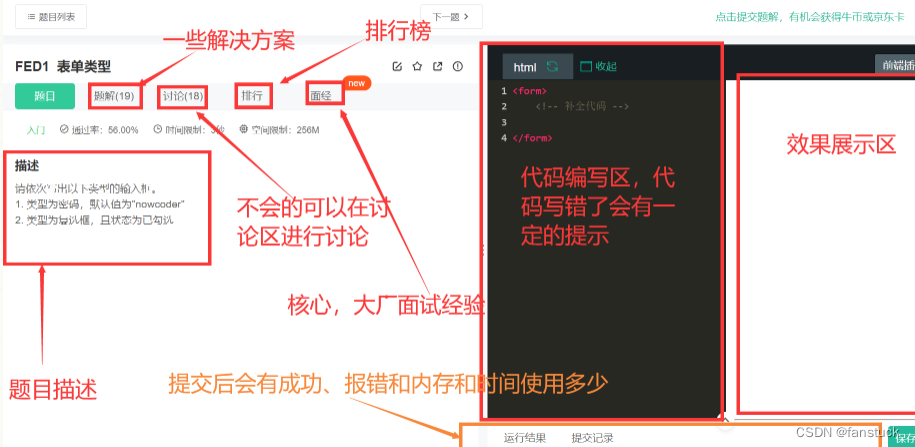# 一、AI1 鸢尾花分类_1

## 描述：

train_and_predict函数接收三个参数:

• train_input_features—二维NumPy数组，其中每个元素都是一个数组，它包含:萼片长度、萼片宽度、花瓣长度和花瓣宽度。

• train_outputs—一维NumPy数组，其中每个元素都是一个数字，表示在train_input_features的同一行中描述的鸢尾花种类。0表示鸢尾setosa，1表示versicolor，2代表Iris virginica。

• prediction_features—二维NumPy数组，其中每个元素都是一个数组，包含:萼片长度、萼片宽度、花瓣长度和花瓣宽度。

• 该函数使用train_input_features作为输入数据，使用train_outputs作为预期结果来训练分类器。请使用训练过的分类器来预测prediction_features的标签，并将它们作为可迭代对象返回(如list或numpy.ndarray)。结果中的第n个位置是prediction_features参数的第n行。

## 解答：

很基础的分类应用场景，指定了sklearn库，那么我选择用随机森林来解答：

``````import numpy as np
from sklearn import datasets
from sklearn.model_selection import train_test_split
from sklearn import metrics
from sklearn.ensemble import RandomForestClassifier

def train_and_predict(train_input_features, train_outputs, prediction_features):

#code start here
rfc = RandomForestClassifier(random_state=0)  #随机森林
rfc = rfc.fit(train_input_features,train_outputs)#训练
prediction_result=rfc.predict(prediction_features)
return prediction_result
#code end here

X_train, X_test, y_train, y_test = train_test_split(iris.data, iris.target,\
test_size=0.3, random_state=0)

y_pred = train_and_predict(X_train, y_train, X_test)

if y_pred is not None:

#code start here
print(metrics.accuracy_score(y_test, y_pred))

#code end here``````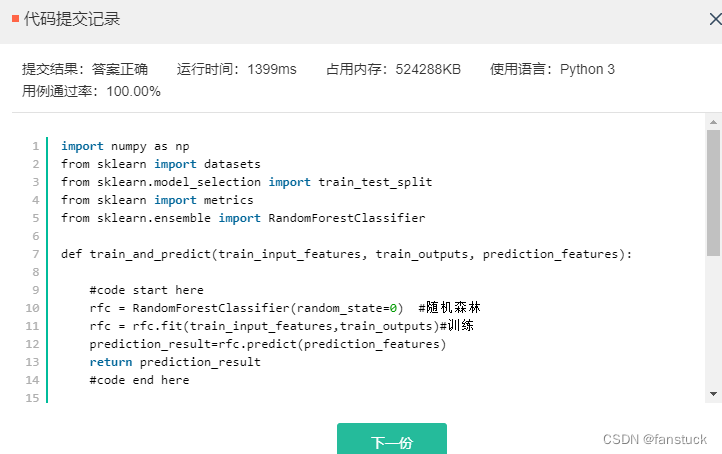# 二、AI2 鸢尾花分类_2

我使用的logistic回归分类器，想要详细了解的可以去：Logistic模型原理详解以及Python项目实现

## 描述

机器学习库 sklearn 自带鸢尾花分类数据集，分为四个特征和三个类别，其中这三个类别在数据集中分别表示为 0, 1 和 2，请实现 transform_three2two_cate 函数的功能，该函数是一个无参函数，要求将数据集中 label 为 2 的数据进行移除，也就是说仅保留 label 为 0 和为 1 的情况，并且对 label 为 0 和 1 的特征数据进行保留，返回值为 numpy.ndarray 格式的训练特征数据和 label 数据，分别为命名为 new_feat 和 new_label。

然后在此基础上，实现 train_and_evaluate 功能，并使用生成的 new_feat 和 new_label 数据集进行二分类训练，限定机器学习分类器只能从逻辑回归和决策树中进行选择，将训练数据和测试数据按照 8:2 的比例进行分割。

要求输出测试集上的 accuracy_score，同时要求 accuracy_score 要不小于 0.95。

## 解答

注意题目描述，使用numpy函数即可：

``````import numpy as np
from sklearn import datasets
from sklearn.model_selection import train_test_split
from sklearn.preprocessing import MinMaxScaler
from sklearn.linear_model import LogisticRegression
from sklearn.metrics import f1_score,roc_auc_score,accuracy_score
from sklearn.tree import DecisionTreeClassifier

def transform_three2two_cate():
#其中data特征数据的key为data，标签数据的key为target
#需要取出原来的特征数据和标签数据，移除标签为2的label和特征数据，返回值new_feat为numpy.ndarray格式特征数据，
#new_label为对应的numpy.ndarray格式label数据
#需要注意特征和标签的顺序一致性，否则数据集将混乱
#code start here
index_2=np.where(np.array(data.target==2))
new_feat=np.delete(data.data,index_2,0)
new_label=np.delete(data.target,index_2)
#code end here
return new_feat,new_label

def train_and_evaluate():
data_X,data_Y = transform_three2two_cate()
train_x,test_x,train_y,test_y = train_test_split(data_X,data_Y,test_size = 0.2)
#已经划分好训练集和测试集，接下来请实现对数据的训练
#code start here
classifier = LogisticRegression(solver='liblinear',C=100)
classifier.fit(train_x, train_y)
y_predict=classifier.predict(test_x)
#code end here
#注意模型预测的label需要定义为 y_predict，格式为list或numpy.ndarray
print(accuracy_score(y_predict,test_y))

if __name__ == "__main__":
train_and_evaluate()
#要求执行train_and_evaluate()后输出为：
#1、{0,1}，代表数据label为0和1
#2、测试集上的准确率分数，要求>0.95``````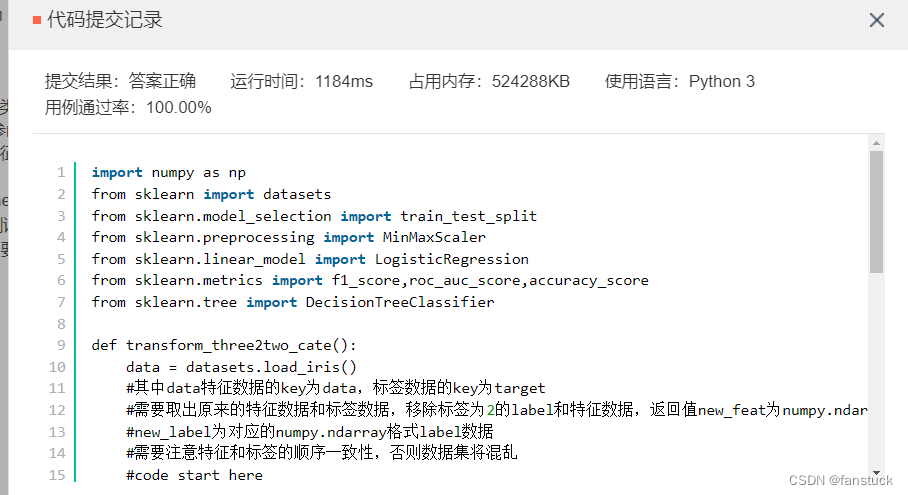# 三、 AI3 决策树的生成与训练-信息熵的计算

想要完整了解决策树算法的可以去：决策树(Decision Tree）算法详解及python实现

## 描述

决策树是非常经典的机器学习模型，以决策树为基模型的集成学习模型（XGBoost、GBDT 等）在工业界得到了极为广泛的应用。决策树有三种常见的启发式生成标准，信息增益就是其中之一。计算某一特征的信息增益主要分为两步，第一步是计算数据集的信息熵，信息熵可以表示为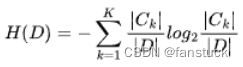，其中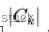代表的是属于某一类的样本个数，D 是整个数据集的样本数量，K 为类别数量。第二步是根据信息熵计算每个特征的经验条件熵。特征的信息增益即为信息熵和经验条件熵的差。现有一数据集，有 4 个特征，分别为教育程度、是否有车、是否有正式工作和征信情况，通过这 4 个特征决策是否予以审批信用卡，数据已经通过 dataSet 给出。其中 dataSet 每行的前 4 列依次代表上述特征的取值，最后一列代表对应的 label 标签。

要求实现 calcInfoEnt 功能，数据集从当前路径下 dataSet.csv读取，计算在给定数据集的情况下，数据集的信息熵，信息熵用 infoEnt 进行表示，数据类型为 float，将 infoEnt 作为函数返回值。计算逻辑参考题目描述中给出的公式。

其中dataSet.csv的示例数据集如下所示：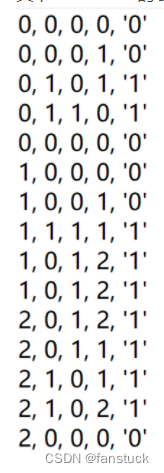## 解答

我在上篇决策树文章中已经实现了熵的计算：

``````# -*- coding: UTF-8 -*-
from math import log
import pandas as pd

def calcInfoEnt(dataSet):
numEntres = len(dataSet)
#code start here
labelCounts = {} #创建记录不同分类标签结果多少的字典
#为所有可能分类保存
#该字典key：label value:label的数目
for featVec in dataSet:
currentLabel = featVec[-1]
if currentLabel not in labelCounts.keys():
labelCounts[currentLabel] = 0
labelCounts[currentLabel] += 1
infoEnt = 0.0
for key in labelCounts:
prob = float(labelCounts[key])/numEntres #标签发生概率p(xi)的值
infoEnt -= prob * log(prob,2)
return infoEnt
#code end here
#返回值 infoEnt 为数据集的信息熵，表示为 float 类型

if __name__ == '__main__':
print(calcInfoEnt(dataSet))
#输出为当前数据集的信息熵``````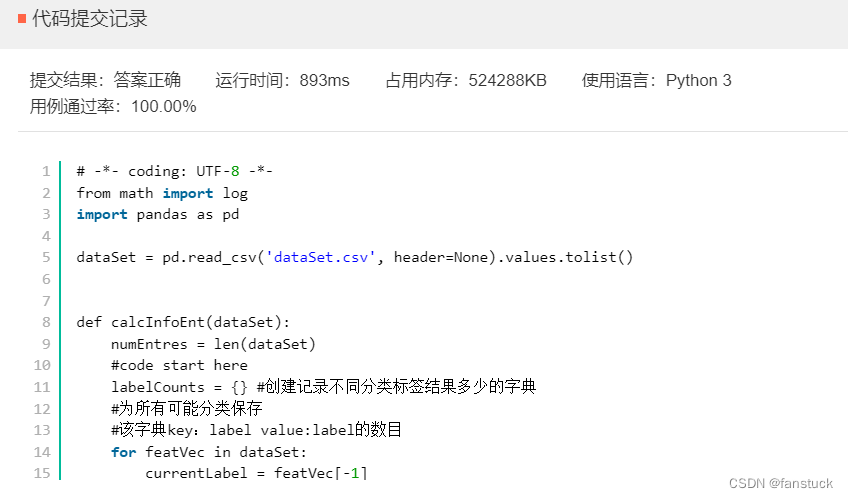# 四、AI4 决策树的生成与训练-信息增益

## 描述

决策树有三种常见的启发式生成算法，信息增益就是其中之一。计算某一特征的信息增益主要分为两步，第一步是计算数据集的信息熵，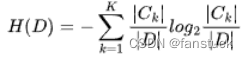第二步是计算每个特征的信息增益，特征 A 对于数据集 D 的经验条件熵可以表示为：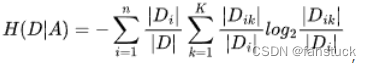其中CK代表的是属于某一类的样本个数，D 是整个数据集的样本数量，根据某一特征不同取值可以将数据划分为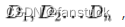其中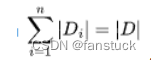K 为类别的数目，某一特征的信息增益即为信息熵和经验条件熵的差

现有一数据集，有 4 个特征，分别为教育程度、是否有车、是否有正式工作和征信情况，通过这 4 个特征决策是否予审批信用卡，数据都已经通过 dataSet 给出，其中 dataSet 每行的前 4 列依次代表上述特征，最后一列代表对应的 label。

实现 calc_max_info_gain 功能，该函数的输入是一个二维数组 dataSet（从当前路径dataSet.csv中读取），要求在给定数据集的情况下，计算所有特征中信息增益最大的特征对应的索引和相应的信息增益值，结果以 list 形式返回，list 长度为2，第一个元素为特征的索引，数据类型为 int，比如教育程度是的索引是 0，是否有车是 1；第二个元素是该特征对应的信息增益，数据类型为 float，最后系统会将该 list 进行输出，在代码部分中，该 list 用 max_info_gain 进行表示。

其中dataSet.csv的示例数据如下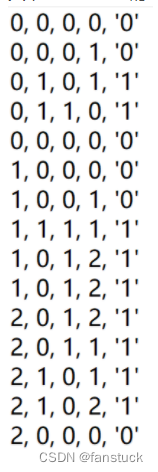## 选择最优划分算法

如何就是决策树的重点，如何选择最优的划分方式，也就是选择信息增益最大化的方式，通过for循环对不同的特征值进行划分，计算每种方式的信息熵，然后取得最大信息增益划分方式，计算最好的信息增益，返回最好特征划分的索引值。决策树(Decision Tree）算法详解及python实现

``````# -*- coding: UTF-8 -*-
from math import log
import pandas as pd

#给定一个数据集，calcInfoEnt可以用于计算一个数据集的信息熵，可直接调用
#也可不使用，通过自己的方式计算信息增益
def calcInfoEnt(data):
numEntres = len(data)
labelcnt = {} #用于统计正负样本的个数
for item in data:
if item[-1] not in labelcnt:
labelcnt[item[-1]] = 0
labelcnt[item[-1]] += 1
infoEnt = 0.0
for item in labelcnt: #根据信息熵的公式计算信息熵
curr_info_entr = float(labelcnt[item]) / numEntres
infoEnt = infoEnt - curr_info_entr * log(curr_info_entr,2)
return infoEnt
#返回值 infoEnt 为数据集的信息熵

#给定一个数据集，用于切分一个子集，可直接用于计算某一特征的信息增益
#也可不使用，通过自己的方式计算信息增益
#dataSet是要划分的数据集，i 代表第i个特征的索引index
#value对应该特征的某一取值
def create_sub_dataset(dataSet, i, value):
res = []
for item in dataSet:
if item[i] == value:
curr_data = item[:i] + item[i+1:]
res.append(curr_data)
return res

def calc_max_info_gain(dataSet):#计算所有特征的最大信息增益，dataSet为给定的数据集
n = len(dataSet)-1  # n 是特征的数量，-1 的原因是最后一列是分类标签
total_entropy = calcInfoEnt(dataSet)#整体数据集的信息熵
max_info_gain =[0,0.0]#返回值初始化
#code start here
bestFeature = -1
for i in range(n):
#创建唯一的分类标签列表
featList = [example[i] for example in dataSet]
uniqueVals = set(featList) #建立列表同特征下不同回答
newEntropy = 0.0
#计算每种划分方式的信息熵
for value in uniqueVals:
subDataSet = create_sub_dataset(dataSet,i,value) #划分
prob = len(subDataSet)/float(len(dataSet)) #同特征下不同回答所占总回答比率
newEntropy += prob * calcInfoEnt(subDataSet) #该特征划分下的信息熵
infoGain = total_entropy - newEntropy #信息增益
if ( infoGain > max_info_gain ):
max_info_gain =infoGain
max_info_gain=i
bestFeature = i
#code end here
return max_info_gain
if __name__ == '__main__':
info_res = calc_max_info_gain(dataSet)
print("信息增益最大的特征索引为：{0},对应的信息增益为{1}".format(info_res,info_res))``````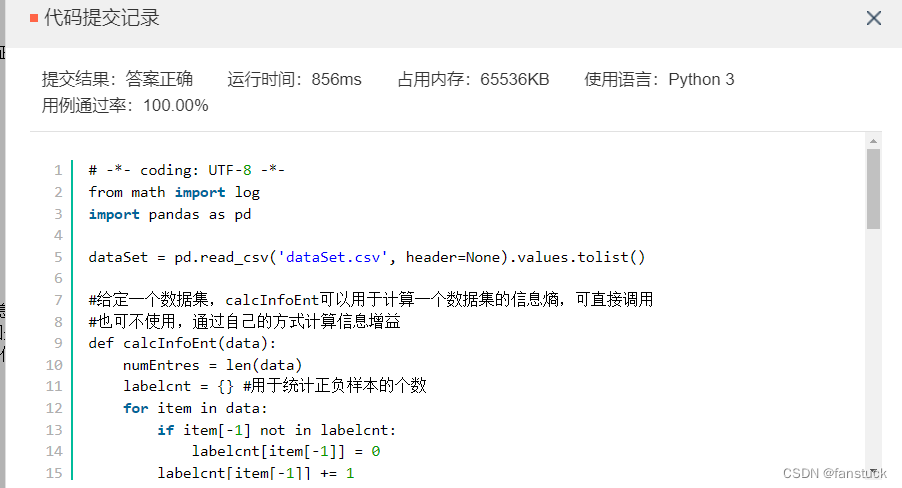# 五、AI5 使用梯度下降对逻辑回归进行训练

题目还是太少了一下就做完了，希望牛客再出点题目，不够做。

## 描述

逻辑回归是机器学习领域中被广泛使用的经典模型。理解和正确使用逻辑回归对于机器学习的实际应用至关重要。逻辑回归通常使用极大似然的思想构建损失函数，并且在此基础上使用梯度下降进行求解。通过求解极大似然思想表示的逻辑回归损失函数对于参数 θ的梯度，可以得到参数的更新公式为：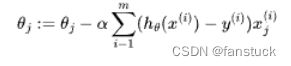，其中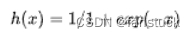请根据上述给定的信息完成接下来的代码补全。

从当前路径下读取数据集dataSet.csv、labels.csv，使用梯度下降的思想训练逻辑回归模型进行训练，需要实现 sigmoid 函数和 gradientDescent 函数。其中，sigmoid 函数只需给出基本的数学实现，gradientDescent 函数的入参是训练数据矩阵 dataMatIn 和 classLabels，其格式均为 np.matrix，matrix 中的特征有3 维度，因此返回值为经过迭代以后的参数矩阵，要求格式为 np.matrix 格式，维度为 3 * 1，分别代表了每一维特征的权重。

其中dataSet.csv示例数据如下：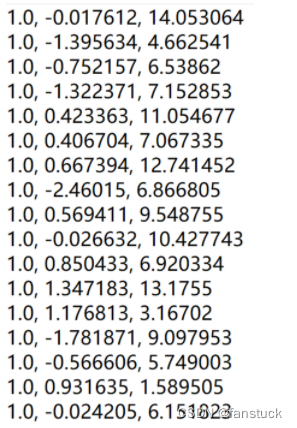lables.csv示例数据集如下：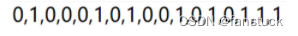## Logistic模型原理详解以及Python项目实现

大家有时间看看这篇文章就好了，这里把题目比较难的点提出来一下：

可以假设为爬山运动，我们总是往向着山顶的方向攀爬，当爬到一定角度以后也会驻足停留下观察自身角度是否是朝着山顶的角度上攀爬。并且我们需要总是指向攀爬速度最快的方向爬。

关于梯度上升的几个概念：

• 1）步长（learning rate）：步长决定了在梯度下降迭代过程中，每一步沿梯度负方向前进的长度
• 2）特征（feature）：指的是样本中输入部门，比如样本（x0，y0），（x1，y1），则样本特征为x，样本输出为y
• 3）假设函数（hypothesis function）：在监督学习中，为了拟合输入样本，而使用的假设函数，记为。比如对于样本（,）(i=1,2,…n),可以采用拟合函数如下：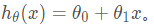• 4）损失函数（loss function）：为了评估模型拟合的好坏，通常用损失函数来度量拟合的程度。损失函数极小化，意味着拟合程度最好，对应的模型参数即为最优参数。在线性回归中，损失函数通常为样本输出和假设函数的差取平方。比如对于样本（,）(i=1,2,…n),采用线性回归，损失函数为：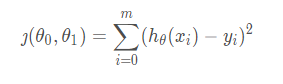其中​表示样本特征x的第i个元素，表示样本输出y的第i个元素， 为假设函数。

• 梯度上升算法的基本思想是：要找到某函数的最大值，最好的方法就是沿着该函数的梯度方向搜寻。我们假设步长为，用向量来表示的话，梯度上升算法的迭代公式如下：

。该公式停止的条件是迭代次数达到某个指定值或者算法达到某个允许的误差范围。

梯度下降也是一样的，无非就是实现公式不同而已。

``````import numpy as np
import pandas as pd
def generate_data():
return datasets, labels
def sigmoid(X):
#补全 sigmoid 函数功能
#code start here
return 1.0/(1+np.exp(-X))
#code end here
alpha = 0.001  # 学习率，也就是题目描述中的 α
iteration_nums = 100  # 迭代次数，也就是for循环的次数
dataMatrix = np.mat(dataMatIn)
labelMat = np.mat(classLabels).transpose()
m, n = np.shape(dataMatrix)  # 返回dataMatrix的大小。m为行数,n为列数。
weight_mat = np.ones((n, 1)) #初始化权重矩阵
#iteration_nums 即为循环的迭代次数
#请在代码完善部分注意矩阵乘法的维度，使用梯度下降矢量化公式
#code start here
for k in range(iteration_nums):
#求当前的sigmoid函数预测概率
h=sigmoid(dataMatrix*weight_mat)
#***********************************************
#此处计算真实类别和预测类别的差值
#对logistic回归函数的对数释然函数的参数项求偏导
error=(h-labelMat)
weight_mat=weight_mat-alpha*dataMatrix.transpose()*error
return weight_mat
#code end here
if __name__ == '__main__':
dataMat, labelMat = generate_data()